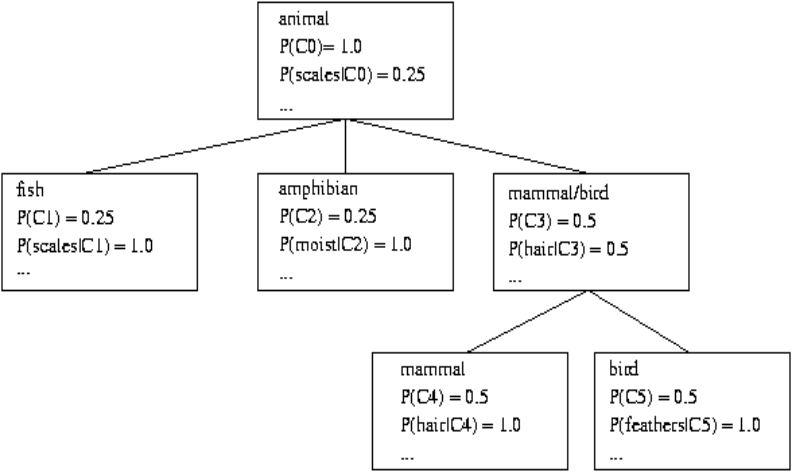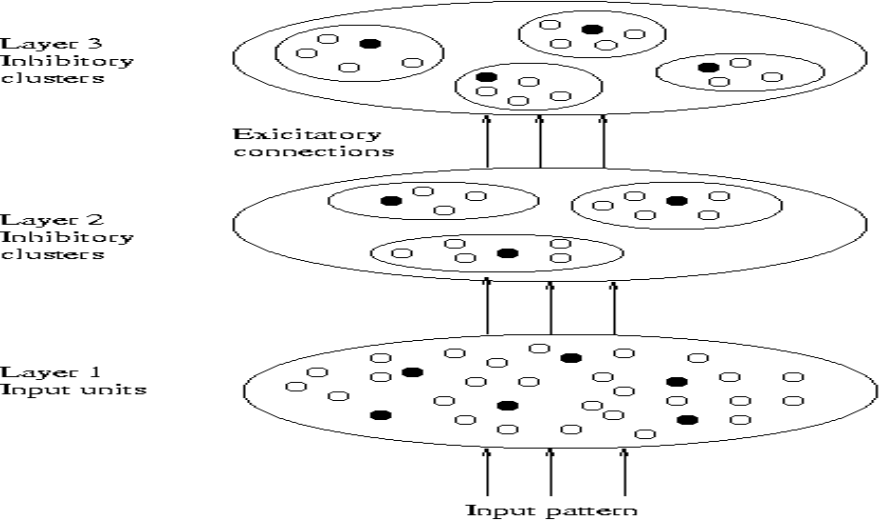# Model-Based Clustering - COBWEB

## Model-Based Clustering

Model-based clustering method is an attempt to optimize the fit between the data and some mathematical models.

It is the Statistical and AI approach.

Model-based clustering works on the intuition that gene expression data originates from a finite mixture of underlying probability distributions (Ramoni et al. 2001).

Each cluster corresponds to a different distribution, and these distributions are assumed to be Gaussians.

The parameters of each distribution (i.e., cluster) are estimated by maximizing the likelihood of the expression data (Hogg and Craig 1994).

The k-means clustering method is a special case of model-based clustering, where all the distributions are assumed to be Gaussians with equal variance.
• Randomly generate the parameters (the parameters would be the mean and standard deviation or covariance matrix) describing each probability distribution (i.e., cluster)
• Repeat until the parameters of each distribution converge
• For each gene, estimate the probability that the gene's expression pattern was generated from each of the distributions.

• For each distribution, estimate the parameters of the distribution to maximize the likelihood of the expression data given the probability that each gene was generated from the distribution.
• Assign each gene to the distribution which generates the gene's expression profile with maximum probability
Model-based clustering has the advantage of providing the probability that each gene belongs in each cluster.

However, model-based clustering operates under the assumption that expression data comes from particular probability distributions, which may not be a reasonable assumption for many microarray data sets.

### Conceptual clustering

Conceptual clustering is a form of clustering in machine learning.

It produces a classification scheme for a set of unlabeled objects and finds characteristic description for each concept (class).

## COBWEB (Fisher’87)

COBWEB is a popular a simple method of incremental conceptual learning.

It creates a hierarchical clustering in the form of a classification tree.

Each node refers to a concept and contains a probabilistic description of that concept.

## Classification Tree## Limitations of COBWEB

The assumption that the attributes are independent of each other is often too strong because correlation may exist.

It is not suitable for clustering large database data – skewed tree and expensive probability distributions.

Some of the other methods alike COBWEB are:

CLASSIT
• It is an extension of COBWEB for incremental clustering of continuous data.
• It suffers similar problems as COBWEB.

AutoClass (Cheeseman and Stutz, 1996)
• It uses Bayesian statistical analysis to estimate the number of clusters.
• It has been popular in the industry.

## Other Model-Based Clustering Methods

Neural network approaches
• It represents each cluster as an exemplar, acting as a “prototype” of the cluster.
• Then new objects are distributed to the cluster whose exemplar is the most similar according to some distance measure.
Competitive learning
• It involves a hierarchical architecture of several units (neurons).
• Neurons compete in a “winner-takes-all” fashion for the object currently being presented.## Self-Organizing Feature Maps

Clustering is also performed by having several units competing for the current object.

The unit whose weight vector is closest to the current object wins.

The winner and its neighbors learn by having their weights adjusted.

SOMs are believed to resemble processing that can occur in the brain.

Useful for visualizing high-dimensional data in 2-D or 3-D space.

## Summary

Model-based clustering -> It is one of the methods of the clustering process which is an attempt to optimize the fit between the data and some mathematical models.

Subscribe us for more content on Data.